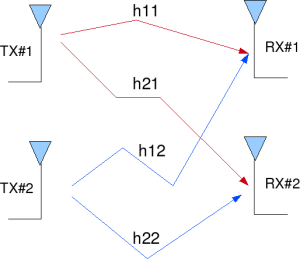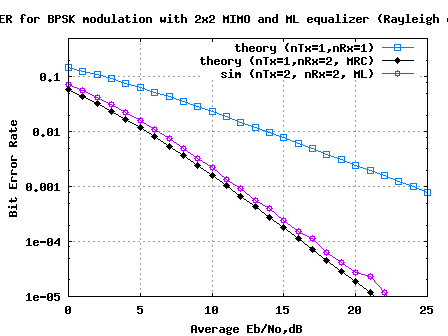# MIMO with ML equalization

We have discussed quite a few receiver structures for a 2×2 MIMO channel namely,

(b) Minimum Mean Square Error (MMSE) equalization

From the above receiver structures, we saw that MMSE equalisation with optimally ordered Successive Interference Cancellation gave the best performance. In this post, we will discuss another receiver structure called Maximum Likelihood (ML) decoding which gives us an even better performance. We will assume that the channel is a flat fading Rayleigh multipath channel and the modulation is BPSK.

## 2×2 MIMO channel

In a 2×2 MIMO channel, probable usage of the available 2 transmit antennas can be as follows:

1. Consider that we have a transmission sequence, for example

2. In normal transmission, we will be sending in the first time slot, in the second time slot, and so on.

3. However, as we now have 2 transmit antennas, we may group the symbols into groups of two. In the first time slot, send and from the first and second antenna. In second time slot, send and from the first and second antenna, send and in the third time slot and so on.

4. Notice that as we are grouping two symbols and sending them in one time slot, we need only time slots to complete the transmission – data rate is doubled !5. This forms the simple explanation of a probable MIMO transmission scheme with 2 transmit antennas and 2 receive antennas.Figure: 2 Transmit 2 Receive (2×2) MIMO channel

Let us now try to understand the math for extracting the two symbols which interfered with each other. In the first time slot, the received signal on the first receive antenna is,

.

.

where

, are the received symbol on the first and second antenna respectively,

is the channel from transmit antenna to receive antenna,

is the channel from transmit antenna to receive antenna,

is the channel from transmit antenna to receive antenna,

is the channel from transmit antenna to receive antenna,

, are the transmitted symbols and

is the noise on receive antennas.

We assume that the receiver knows , , and . The receiver also knows and . The unknown s are and .

For convenience, the above equation can be represented in matrix notation as follows:

.

Equivalently,

## Other Assumptions

1. The channel is flat fading – In simple terms, it means that the multipath channel has only one tap. So, the convolution operation reduces to a simple multiplication. For a more rigorous discussion on flat fading and frequency selective fading, may I urge you to review Chapter 15.3 Signal Time-Spreading from [DIGITAL COMMUNICATIONS: SKLAR]2. The channel experience by each transmit antenna is independent from the channel experienced by other transmit antennas.

3. For the transmit antenna to receive antenna, each transmitted symbol gets multiplied by a randomly varying complex number . As the channel under consideration is a Rayleigh channel, the real and imaginary parts of are Gaussian distributed having mean and variance .

4. The channel experienced between each transmit to the receive antenna is independent and randomly varying in time.

5. On the receive antenna, the noise has the Gaussian probability density function with

with and .

7. The channel is known at the receiver.

The Maximum Likelihood receiver tries to find which minimizes,

Since the modulation is BPSK, the possible values of is +1 or -1 Similarly also take values +1 or -1. So, to find the Maximum Likelihood solution, we need to find the minimum from the all four combinations of and .

The estimate of the transmit symbol is chosen based on the minimum value from the above four values i.e

if the minimum is ,

if the minimum is ,

if the minimum is and
if the minimum is .

## Simulation Model

The Matlab/Octave script performs the following

(a) Generate random binary sequence of +1’s and -1’s.

(b) Group them into pair of two symbols and send two symbols in one time slot

(c) Multiply the symbols with the channel and then add white Gaussian noise.

(d) Find the minimum among the four possible transmit symbol combinations

(e) Based on the minimum chose the estimate of the transmit symbol

(h) Repeat for multiple values of and plot the simulation and theoretical results.BER plot 2x2 MIMO Rayleigh channel with Maximum Likelihood equalisation

FIgure: BER plot 2×2 MIMO Rayleigh channel with Maximum Likelihood equalisation

## Summary

1. The results for 2×2 MIMO with Maximum Likelihood (ML) equalization helped us to achieve a performance closely matching the 1 transmit 2 receive antenna Maximal Ratio Combining (MRC) case.

2. If we use a higher order constellation like 64QAM, then computing Maximum Likelihood equalization might become prohibitively complex. With 64QAM and 2 spatial stream we need to find the minimum from combinations ! In such scenarios we might need to employ schemes like sphere decoding which helps to reduce the complexity.

## References

[DIG-COMM-BARRY-LEE-MESSERSCHMITT] Digital Communication: Third Edition, by John R. Barry, Edward A. Lee, David G. Messerschmitt[WIRELESS-TSE, VISWANATH]Fundamentals of Wireless Communication, David Tse, Pramod Viswanath%%%%%%%%%%%%%%%%%%%%%%%%%%%%%%%%%%%%%%%%%%%%%%%%%%%%%%%%%%%%
% The file may not be re-distributed without explicit authorization
% from Krishna Pillai.
% Checked for proper operation with Octave Version 3.0.0
% Author        : Krishna Pillai
% Email         : krishna@dsplog.com
% Version       : 1.0
% Date          : 14th December 2008
% %%%%%%%%%%%%%%%%%%%%%%%%%%%%%%%%%%%%%%%%%%%%%%%%%%%%%%%%%

% Script for computing the BER for BPSK modulation in a
% Rayleigh fading channel with 2 Tx, 2Rx MIMO channel
% Maximum Likelihood equalization

clear
N = 10^6; % number of bits or symbols
Eb_N0_dB = [0:25]; % multiple Eb/N0 values
nTx = 2;
nRx = 2;
for ii = 1:length(Eb_N0_dB)

% Transmitter
ip = rand(1,N)>0.5; % generating 0,1 with equal probability
s = 2*ip-1; % BPSK modulation 0 -> -1; 1 -> 0
sMod = kron(s,ones(nRx,1)); %
sMod = reshape(sMod,[nRx,nTx,N/nTx]); % grouping in [nRx,nTx,N/NTx ] matrix

h = 1/sqrt(2)*[randn(nRx,nTx,N/nTx) + j*randn(nRx,nTx,N/nTx)]; % Rayleigh channel
n = 1/sqrt(2)*[randn(nRx,N/nTx) + j*randn(nRx,N/nTx)]; % white gaussian noise, 0dB variance

% Channel and noise Noise addition
y = squeeze(sum(h.*sMod,2)) + 10^(-Eb_N0_dB(ii)/20)*n;

% ----------------------------
% if [s1 s2 ] = [+1,+1 ]
sHat1 = [1 1];
sHat1 = repmat(sHat1,[1 ,N/2]);
sHat1Mod = kron(sHat1,ones(nRx,1));
sHat1Mod = reshape(sHat1Mod,[nRx,nTx,N/nTx]);
zHat1 = squeeze(sum(h.*sHat1Mod,2)) ;
J11 = sum(abs(y - zHat1),1);

% if [s1 s2 ] = [+1,-1 ]
sHat2 = [1 -1];
sHat2 = repmat(sHat2,[1 ,N/2]);
sHat2Mod = kron(sHat2,ones(nRx,1));
sHat2Mod = reshape(sHat2Mod,[nRx,nTx,N/nTx]);
zHat2 = squeeze(sum(h.*sHat2Mod,2)) ;
J10 = sum(abs(y - zHat2),1);

% if [s1 s2 ] = [-1,+1 ]
sHat3 = [-1 1];
sHat3 = repmat(sHat3,[1 ,N/2]);
sHat3Mod = kron(sHat3,ones(nRx,1));
sHat3Mod = reshape(sHat3Mod,[nRx,nTx,N/nTx]);
zHat3 = squeeze(sum(h.*sHat3Mod,2)) ;
J01 = sum(abs(y - zHat3),1);

% if [s1 s2 ] = [-1,-1 ]
sHat4 = [-1 -1];
sHat4 = repmat(sHat4,[1 ,N/2]);
sHat4Mod = kron(sHat4,ones(nRx,1));
sHat4Mod = reshape(sHat4Mod,[nRx,nTx,N/nTx]);
zHat4 = squeeze(sum(h.*sHat4Mod,2)) ;
J00 = sum(abs(y - zHat4),1);

% finding the minimum from the four alphabet combinations
rVec = [J11;J10;J01;J00];
[jj dd] = min(rVec,[],1);

% mapping the minima to bits
ref = [1 1; 1 0; 0 1; 0 0 ];
ipHat = zeros(1,N);
ipHat(1:2:end) = ref(dd,1);
ipHat(2:2:end) = ref(dd,2);

% counting the errors
nErr(ii) = size(find([ip- ipHat]),2);

end

simBer = nErr/N; % simulated ber
EbN0Lin = 10.^(Eb_N0_dB/10);
theoryBer_nRx1 = 0.5.*(1-1*(1+1./EbN0Lin).^(-0.5));
p = 1/2 - 1/2*(1+1./EbN0Lin).^(-1/2);
theoryBerMRC_nRx2 = p.^2.*(1+2*(1-p));

close all
figure
semilogy(Eb_N0_dB,theoryBer_nRx1,'bp-','LineWidth',2);
hold on
semilogy(Eb_N0_dB,theoryBerMRC_nRx2,'kd-','LineWidth',2);
semilogy(Eb_N0_dB,simBer,'mo-','LineWidth',2);
axis([0 25 10^-5 0.5])
grid on
legend('theory (nTx=1,nRx=1)', 'theory (nTx=1,nRx=2, MRC)', 'sim (nTx=2, nRx=2, ML)');
xlabel('Average Eb/No,dB');
ylabel('Bit Error Rate');
title('BER for BPSK modulation with 2x2 MIMO and ML equalizer (Rayleigh channel)');Sam Iliffe 说:
2019年4月02日 14:48

I have deleted before to read the post carefully under the depending terms of the logically parts as well. Now feeling regret that without the opening of the topic on the writing services I have typed the whole comment frequently.(输入验证码)
or Ctrl+Enter